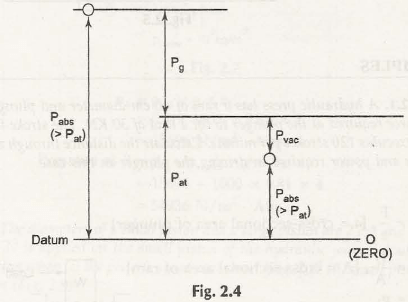Atmospheric Pressure:

Atmospheric Pressure is the pressure exerted by weight of atmosphere on different fluids. At sea level, its standard value is considered as 101.325 Pa.

In fluid mechanics, certain other phenomena are also considered such as gauge pressure, vacuum pressure and absolute pressure as shown in Fig. 2.4P = Total Pressure

Pabs = Absolute Pressure

Pg = Gauge Pressure

Pat = Atmospheric Pressure

Pvac= Vacuum Pressure

Absolute Pressure:

The pressure which is measured from absolute zero level and below vacuum pressure is called absolute pressure.

GaugePressure:

The pressure above atmospheric pressure is the gauge pressure.

Vacuum Pressure:

The below atmospheric pressure is called vacuum pressure.

Mathematically, these pressures can be formulated as,

• Absolute Pressure, Pabs =Guage Pressure, Pg +Atmospheric Pressure, Pat
• Vacuum Pressure, Pvac= Atmospheric Pressure, Pat–Absolute Pressure, Pabs

Links of Next Mechanical Engineering Topics:-### Customer Reviews

My Homework Help
Rated 5.0 out of 5 based on 510 customer reviews at